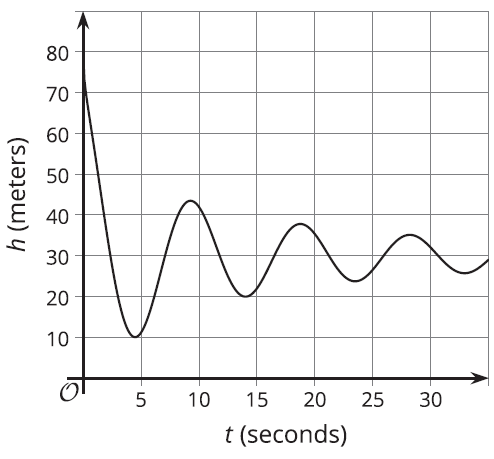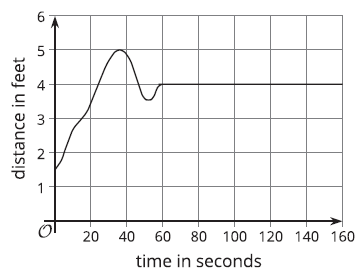# A.4.6.3 The Jump

In a bungee jump, the height of the jumper is a function of time since the jump begins. Function h defines the height, in meters, of a jumper above a river, t seconds since leaving the platform. Here is a graph of function h, followed by five expressions or equations and five graphical features.Expression/Equations: h(0) h(t)=0 h(4) h(t)=80 h(t)=45 Features: first dip in the graph vertical intercept first peak in the graph horizontal intercept maximum 1. Match each description about the jump to a corresponding expression or equation and to a feature on the graph. One expression or equation does not have a matching verbal description. Its corresponding graphical feature is also not shown on the graph. Interpret that expression or equation in terms of the jump and in terms of the graph of the function. Record your interpretation in the last row of the table.

2. Use the graph to: a. estimate h(0) and h(4) b. estimate the solutions to h(t)=45 and h(t)=0

Function b gives the vertical distance (or the height) of a bee from the ground as a function of time, t.The outputs of both functions are measured in feet and the inputs are both measured in seconds. Identify the following features on the graph and interpreting them in terms of the situation:

• vertical intercept
• horizontal intercept
• maximum
• minimum
• intervals where the function is increasing
• intervals where the function is decreasing
• intervals where the function is staying constant
• solution to b(t)=3.5

How can you tell that a point on the graph is a maximum, a minimum, or neither?”

How many intercepts can the graph of a function have?